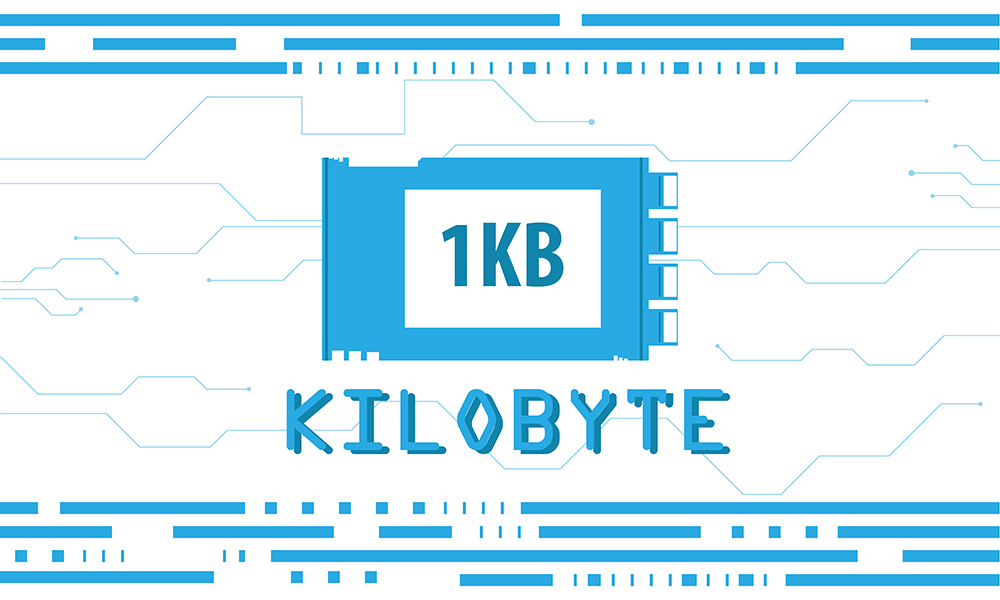When you want to specify which amount of data a hard disk or DVD may contain, or to indicate the amount of its memory, it is very likely that you will use a unit named kilobyte.

One kilobyte is equal to an amount that is by 210 larger than a byte, which is 1 024 bytes. However, a kilobyte can also have a value of 1,000 bytes, which often confuses many users, and the explanation is actually very simple, it is only necessary to understand that it is a different presentation system, that is, a so-called decimal and binary system.

Namely, prefix kilo is used when it is necessary to mark a unit that is a thousand times larger than the basic unit, regardless of which unit it is (kilogram, kilobyte, kilometer ...). The best example to understand this is a 1-kilogram measure that corresponds to a weight of 1,000 grams or 1 kilometer, which is the same as 1,000 meters, while 1 kilobyte is designated as a unit that is by 210 larger than a byte.

Also, to fully understand this, it is necessary to understand the difference between bits and bytes. The unit known as the bit is an abbreviation of two English words, Binary and Unity. The bit can have a value of 0 or 1. It means that 1 b is equal to 0, that is, 1 b is equal to 1. On the other hand, byte (shortened B) consists of a total of 8 bits, that is 1 B = 8 b. In the binary system, for example, letter A, which is 1 byte is designated as 01100001, which is a total of 8 bits, or 8 basic units.

With bit and byte, there are kilobit or kilobyte. The kilobit unit is abbreviated as Kb, as opposed to a kilobyte which is abbreviated as KB. This type of writing abbreviations has been adopted on the basis of a proposal by an international non-profit organization whose aim is to improve the technology related to electricity, the IEEE (The Institute of Electrical and Electronics Engineers).

The kilobyte unit, serving its basic purpose, is relatively rare in modern times. This is due to the fact that many computer components, primarily hardware, nowadays have a much larger amount of memory for storing data, so megabytes and gigabytes are more often used. In order to have a better idea of the amount of quantity indicated by this unit, remember that for example, 1 KB takes one paragraph of text in the Notepad program, which contains a total of 1 024 characters.